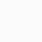Maths And Physics With Pandey Sir Ncert 12th Physics Chapter-4 Force Between Two Parallel Current Carrying Conductors

# Force Between Two Parallel Current Carrying Conductors

Chapter-4|Moving Charges and Magnetism| NCERT 12th Physics:

Force Between Two Parallel Current Carrying Conductors:

Let we consider two parallel infinite long straight conductors.

Note: Two linear parallel conductors carrying current in the same direction attract each other, while carrying in opposite direction they repel to each other.

Definition of Ampere:

1 Ampere: 1 ampere is the current which flow through each of two parallel infinite wires placed at a distance of 1 metre apart produces an attractive or repulsive force per unit length between wire of 2×10⁻⁷ N/m.

## 1 thought on “Force Between Two Parallel Current Carrying Conductors”

1.Psychic in Tampa says:

sir expalin equation 1